# Control System – Bode Plot

Bode plot is a frequency domain technique(used in frequency response analysis) in which magnitude and phase are drawn one below the other. Hence we have two graphs,

Graph 1 : The magnitude (M) of the transfer function as a function of frequency (ω) and

Graph 2 : The phase angle (φ) as a function of frequency (ω)

In graph 1 , magnitude of GH (jω) , the open-loop transfer function, is plotted in decibels on the y-axis while the input frequencies are plotted on x-axis .

In graph 2 , phase angle of GH (jω) plotted on y- axis and the x-axis has the input frequencies in the log scale (similar to graph 1).

Both the above characteristics are plotted on a single semilog paper with the same x-axis scale used for both the graphs.

Standard form for   GH (jω) :

The frequency transfer function can be written in a generalized form as the ratio of polynomials.

GH(s)  =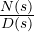=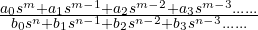This must be factorized to obtain general type,

GH(s)  =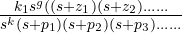We convert the above equation in the time constant form,

GH(s)  =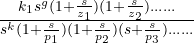We now put s = jω to convert the transfer function to the frequency domain ,

GH (jω)  =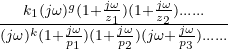Here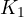is the gain constant for Bode gain. Above equation is the standard form or Bode form. The following is a list of possible factors of GH(jω) ,

• Bode gainor constant factor .
• Poles at origin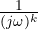of order k.
• Zeros at origin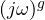of order g.
• Finite simple poles at   (1+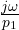)  or  first order poles.
• Finite simple zeros at  (1+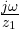)  or  first order zeros.
• Second order poles at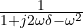• Second order zeros atFrequency domain Specifications :

1. Gain Margin:

It is a measure of relative stability , higher the gain margin higher will be the stability of the machine and is defined as reciprocal of the open loop transfer function, evaluated at phase crossover frequency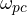which is the frequency at which the phase is  -180. i.e ,

Gain margin :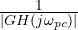where ,   ∠ GH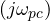=   -180,

Where ‘‘  is the phase crossover frequency.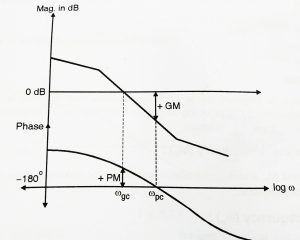1. Phase margin (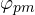) :

Higher the phase margin higher will be the stability of the system .Phase margin is a measure of relative stability and is defined as  180plus the phase angle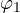of the open loop transfer function at unity gain . That is ,=  [ 180 + arg GH(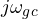)  ] degrees

Where | GH()| = 1  and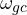is the gain cross over frequency as shown in the above figure.

1. Bandwidth:

The bandwidth of a system is that range of frequencies , over which the system responds satisfactorily. This performance is determined by the application and the characteristics of a particular system.

Often bandwidth of a system defined as a range of frequencies over which the magnitude ratio does not differ by more than – 3 dB from its value at specified frequency. For many feedback control system, this frequency is zero .The bandwidth is in this case equal to cutoff frequency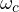.

1. Cutoff frequency () :

The frequency ‘‘ at which the magnitude of closed loop response is 3dB below its zero frequency value is called ‘cut off frequency  ‘  .

1. Cut off Rate :

The cut off rate is the frequency rate at which the magnitude ratio decreases beyond the cut off frequency.

1. Resonance Peak Frequency (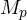) :

The resonance peak, a measure of relative stability , is the maximum value of the magnitude of the closed loop frequency response.

1. Resonant frequency (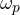) :

The resonant frequency is the frequency at which ‘‘  occurs .

1. Gain crossover frequency :

This is the point on the magnitude plot at which the magnitude of  GH(jω) is unity. It is measured in terms of frequency.

1. Phase margin Angle (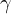) :

This angle is 180plus the negative trigonometrically considered angle of the transfer function at gain crossover frequency.

1. Phase crossover frequency :

This is the frequency at which the phase of GH is  -180.

Relative Stability :

The relative stability of a system is indicated by gain margin and the phase margin in terms of the system’s open loop frequency response . Further, these parameters can be easily determined from the Bode plot of GH(jω). In most cases positive gain and phase margin as defined will ensure the stability of the closed-loop system.

For stable system ,<i.e GM and PM = positive

For unstable system ,>i.e GM and PM = negative

Shown below are the different types of stability obtained using Bode plots:

1. When both GM and PM are positive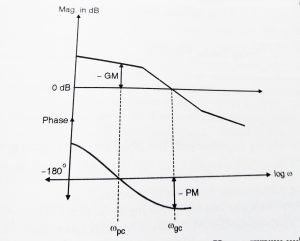2. when both GM and PM is negative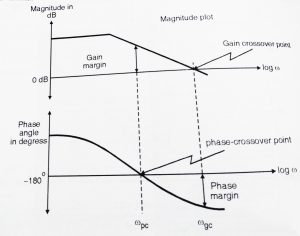When GM is +∞ , the system is absolutely stable and when GM and PM both are zero, the system is marginally stable.

In my next post we will see complete steps for solving Bode Plots(along with an example). Meet you all in my next post.